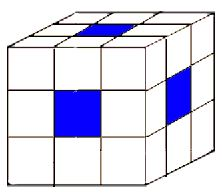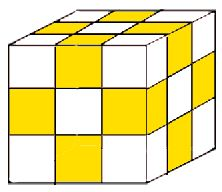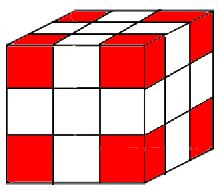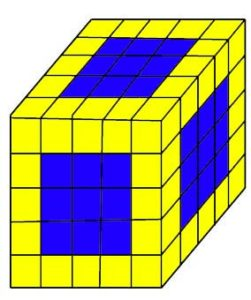0
16856

Cube Questions for CAT Exam

In the CAT Previous Years’ Papers, there have been puzzle based Cube Questions for CAT Exam. Therefore, we have come up with with the concept notes on Cube Questions for CAT. Hence,  Therefore, concept practice on this is utmost importance to not leave any stones unturned.

Any cube has the following characteristic:

• 6 faces
• 12 edges
• 8 corners

UNPAINTED CUBE

Equal Cuts: Each side of the cube is $\left(\frac{1}{n}\right)^{th}$ of the original side if side of the cube is divided into $n$ parts. As a result, by cutting in such a manner, we finally form $n^3$ number of smaller cubes.

Unequal Cuts: If each side of the cube is cut in an unequal manner, such that along the length has L cuts or ‘L+1’ parts, along the breadth, has B cuts or ‘B+1’ parts and along the height has H cuts or ‘H+1’ parts. The total number of pieces formed in this case would be (L+1)(B+1)(H+1)

PAINTED CUBE

A Cube painted on all the faces is cut along length, breadth and height, three types of the cube are obtained.

1. Zero Painted Face: At center of the larger cube
2. Single Painted Face: Face of the larger cube
3. Two Painted Face: Along the edge of the larger cube
4. Three Painted Face: Corner of the larger cube

Single Painted FaceTwo Painted FaceThree Painted FaceIn addition,we have to note that there can be no smaller cube which has all the face painted.

Cube( n x n x n ) Cut into Cubes :

Total Number of Cubes: $n^3$
Zero Painted Face : $(n-2)^3$
Single Painted Face : $6(n-2)^2$
Two Painted Face: $12(n-2)$
Three Painted Face: $8$

Cuboid( p x q x r ) Cut into Cubes :

Total Number of Cubes: $p \times q \times r$
Zero Painted Face : $(p-2) \times (q-2) \times (r-2)$
Single Painted Face : $2(p – 2)(q – 2)+2(q – 2)(r – 2)+2(r – 2)(p – 2)$
Two Painted Face: $4(p-2)+4(q – 2)+4(r – 2)$
Three Painted Face: $8$

So, the above results represent the number of cubes which can be formed by making the equal length cutting. The table below mentions direct results which can come handy in Cube Questions for CAT Exam.

 Number of Sides 0 – Painted Faces 1 – Painted Faces 2 – Painted Faces 3 – Painted Faces Number of Cubes Total Painted Cubes 2 0 0 0 8 8 8 3 1 6 12 8 27 26 4 8 24 24 8 64 56 5 27 54 36 8 125 98 6 64 96 48 8 216 152 7 125 150 60 8 343 218 8 216 216 72 8 512 296 9 343 294 84 8 729 386 10 512 384 96 8 1000 488

Examples on Cube Questions for CAT Exam

Example 1: Find the least number of cuts required which can cut a cube into 24 identical pieces?

Solution : First of all, if we have 24 pieces, factorize the number it into three numbers such that all the factors are greater than 1.

=> 24 = 2 x 2 x 2 x 3

=> 24 = 2 x 3 x 4

Therefore, by using the above mentioned formulas, the number of pieces along different lengths leads to formation of (L+1)(B+1)(H+1) cubes. Hence, by comparing we get L = 1 , B = 2 and H = 3. Therefore, number of cuts is equal to (1 + 2 + 3) cuts or 6 cuts

Example 2: Find the maximum number of smaller pieces with 19 cuts on a cube?

Solution: Since, equal number of cuts in each direction gives the maximum number of pieces. So, we can split 19 cuts into 6,6 and 7.

Therefore, by the number of pieces formed = (6 + 1)(6 + 1)(7 + 1) pieces = 7 * 7 * 8 = 392 pieces

Example 3: A cube of side 20 cm is of blue color with a 4 cm wide yellow strip along all the sides on all the faces. The cube is then cut into 125 smaller equal size cubes

(1) How many cubes have three yellow faces each?

(2) How many cubes have one face blue and an adjacent face yellow?

(3) How many cubes have at least one face colored?

(4) How many cubes have at least two yellow faces each?

First of all, the cube is cut into 125 smaller cubes of equal size. So, we get a stack of cubes in the figure below:Hence, we start by analyzing the figure. 5 horizontal stacked layers are present in the figure

(1): Cubes with corners painted yellow.

Since, all the cubes with painted yellow face are at corner. Therefore, 3 Yellow Painted Face Cubes are 8 in number

(2): One face blue and an adjacent face yellow

Since, there would be no cube having one face blue and an adjacent face yellow as all the yellow painted cubes got paint on at least 2 faces. Therefore, the answer is zero

(3): At least one face colored

Because, the number of cubes with no painted face, $(n−2)^3$ = $(5−2)^3$ =  27

Therefore, there are (125 – 27) = 98 cubes which have at least one face colored.

(4): At least two yellow faces

So, by subtracting the cubes with blue painted face and cubes with no painted face from total number of cubes

Therefore, number of cubes of such types = 125 – (9 x 6) – 27 = 44